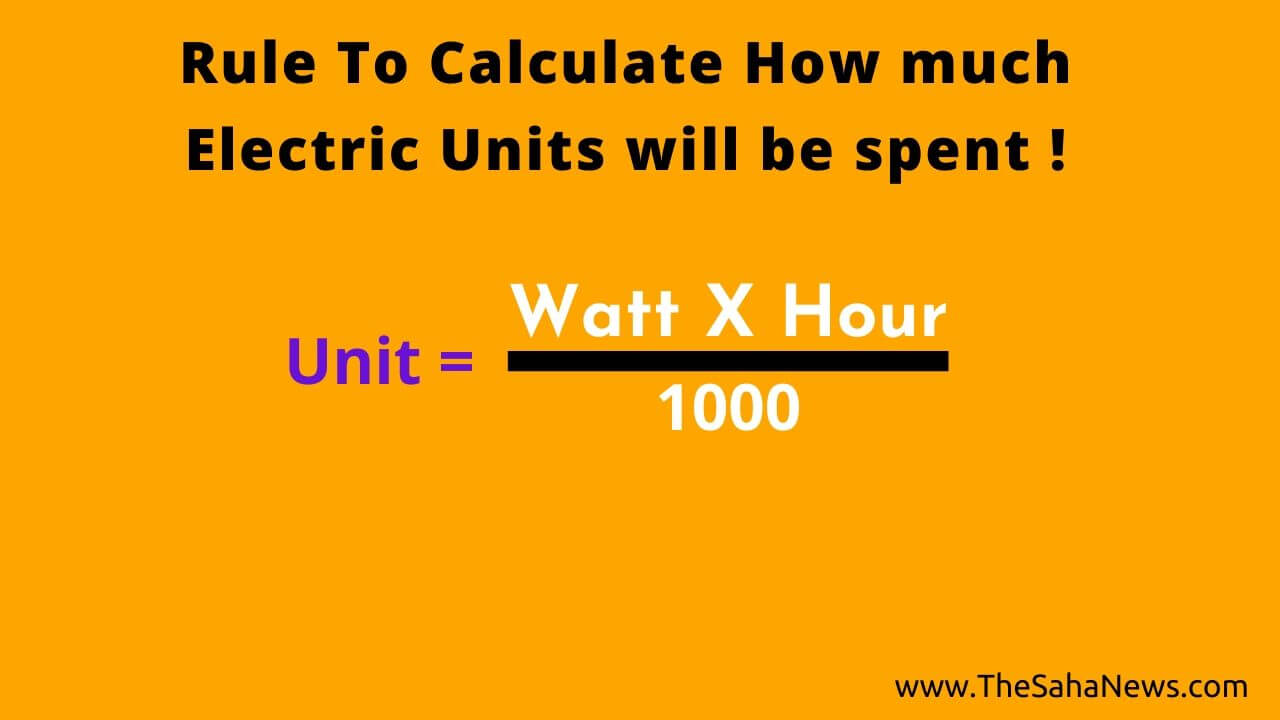Connect with us

How to calculate home electric units usageIf you want to know how much electricity your bulbs, fans, TV, fridge and any other electrical things consuming in your home then you are in the right place. In this post, I will try to teach you how to calculate the bills with a few simple steps.

Know how to calculate how much units it will take for spending electricity with easy techniques:

Rules To Calculate

Watts × Hour ÷ 1000 = Unit

Explanation of the rule

Just use the above rules like multiply the watts(of electrical things that you are using) with the number of hours that are used and then divide them with 1000 & you will get the Unit that consumed.

Example

If you are using 4 bulbs of 9 watt and 3 fans of 50 watt and a TV of 40 watt for 7 hours in a day then how much Unit it will consume for that particular day?

Watts = ( 4×9)+(3×50)+(1×50)
= 36+150+50
=236

Now – Multiply 236 with 7 Hours and divide by 1000.

So, it will be like this – 236×7÷1000 Unit
=1.652 Unit

Conclusion

Using the above rules you can easily calculate your electric units through Watts & hour of usage and then multiply that unit with the exact unit price and you will get the amount consumed by electronic goods.